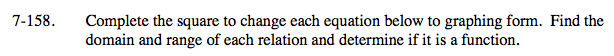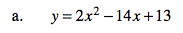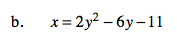### Home > A2C > Chapter 7 > Lesson 7.3.1 > Problem7-158

7-158.13 is not the correct number for a complete square.
Move it to the opposite side of the equation.

y − 13 = 2x2 − 14

Factor out the 2 because it will not make a ncomplete square.n

y − 13 = 2(x2 − 7x)See the steps in part (a).

The graph in part (a) was a y = x2 parabola.
This in an x = y2 parabola.
How does this change the graph and hence the domain and range?

(x − ?)(x − ?) = x2 − 7x + ?2
What number does the ? above need to be?

It needs to be 3.5 (or seven-twelfths).

3.52 needs to be added in the parenthesis.
This is actually 2(3.52) being added on the right side.
Add 2(3.52) to the left side as well.

Factor.

To find the domain and range, think about what the graph looks like.